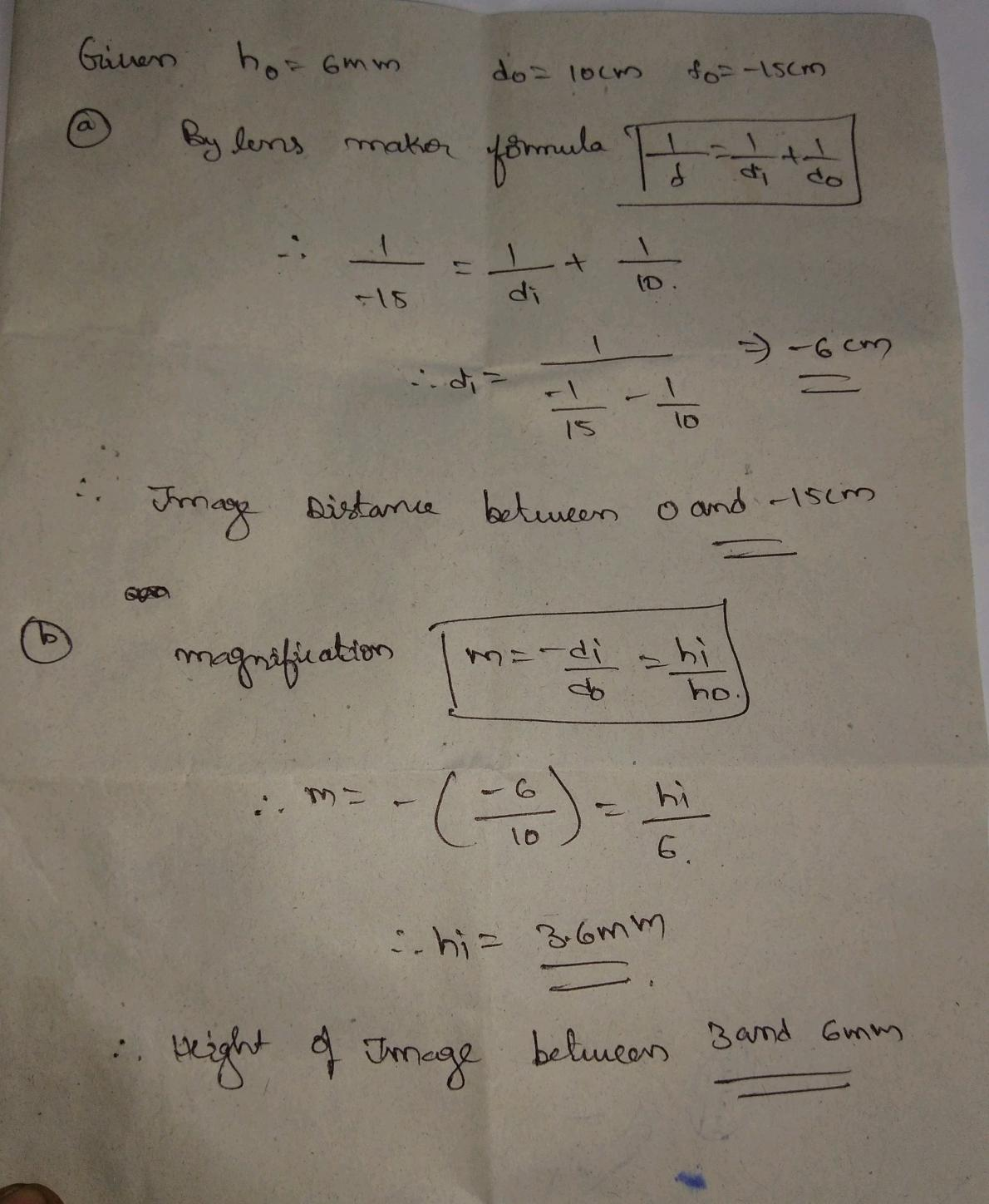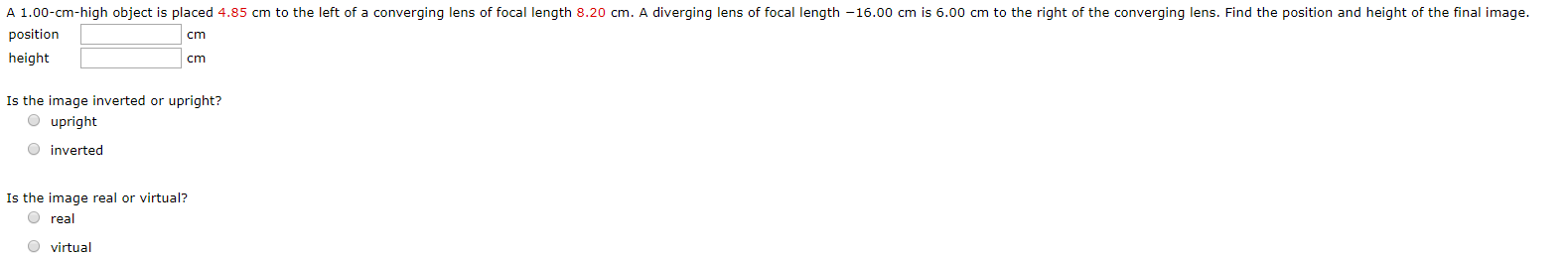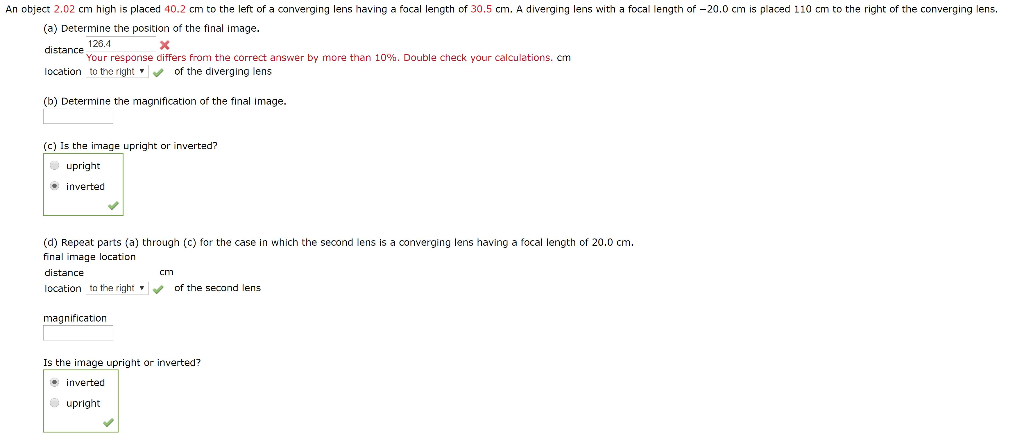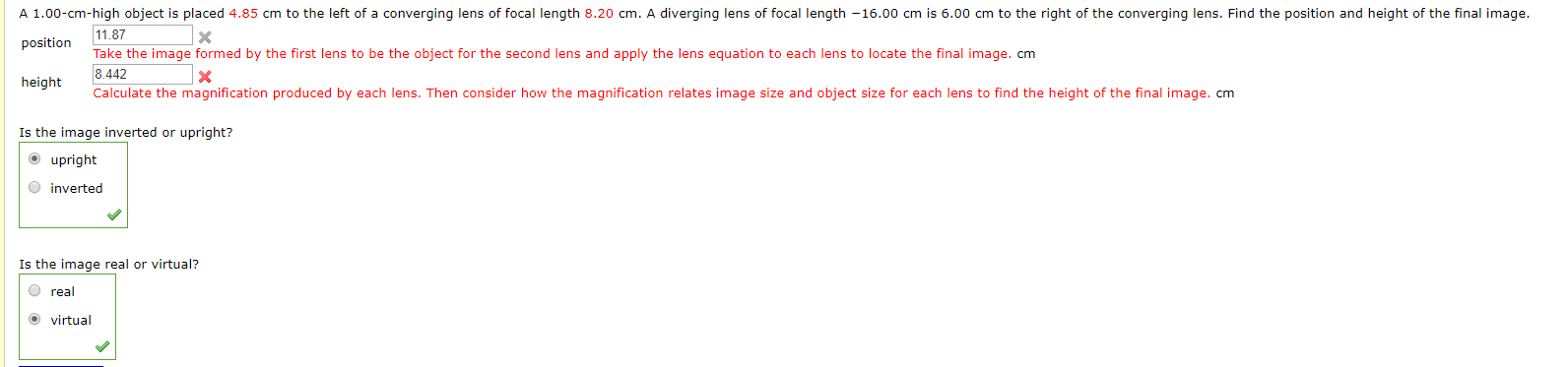# A 6 mm high object is placed 10 cm to the left of a diverging lens...

A 6 mm high object is placed 10 cm to the left of a diverging lens with focal length -15 cm.

1. The image distance will be:

Between 0 and 15 cm

Greater than 15 cm

Between 0 and -15 cm

Less than -15 cm

2. The magnitude of the image height will be:

Between 3 and 6 mm

Greater than 9 mm

Between 6 and 9 mm

Less than 3 mm##### Add Answer of: A 6 mm high object is placed 10 cm to the left of a diverging lens...
Similar Homework Help Questions
• ### A 6 mm high object is placed 48 cm to the left of a converging lens...

A 6 mm high object is placed 48 cm to the left of a converging lens with focal length 12 cm. 1) The image distance will be: Less than -20 cm Between 0 and 20 cm Greater than 20 cm Between 0 and -20 cm 2) The magnitude of the image height will be: Between 6 and 9 mm Between 3 and 6 mm Less than 3 mm Greater than 9 mm A 6 mm high object is placed 10...

• ### A 4 mm high object is placed 5 cm in front of a concave mirror with...

A 4 mm high object is placed 5 cm in front of a concave mirror with a radius of curvature 20 cm. 1)The image distance is: Between -15 cm and zero Less than -15 cm Greater than 15 cm Between 0 and 15 cm 2) The magnitude of the image height will be: Less than 3 mm Between 3 and 6 mm Greater than 9 mm Between 6 and 9 mm 3) The image will be: Virtual and inverted Real...

• ### An object is placed 32.0 cm to the left of a diverging lens with a focal...

An object is placed 32.0 cm to the left of a diverging lens with a focal length of -20.0 cm. A converging lens of focal length of 32.0 cm is placed a distance d to the right of the diverging lens. Find the distance d that the final image is at infinity. ______cm

• ### An object 3 cm tall is placed 15 cm to the left of a diverging lens...

An object 3 cm tall is placed 15 cm to the left of a diverging lens having a focal length f=-30 cm. What is the size of the resulting image?

• ### Use the thin lens equation to solve problems 14 –18. 14. An object is 10 cm...

Use the thin lens equation to solve problems 14 –18. 14. An object is 10 cm high and is placed 20 cm in front of a converging lens of focal length 20 cm. Determine the image distance, the image height and the magnification. 15. An object is 10 cm high and is placed 16 cm in front of a converging lens of focal length 20 cm. Determine the image distance, the image height and the magnification. 16. An object is...

• ### A 4 mm high object is placed 9 cm in front of a convex mirror with...

A 4 mm high object is placed 9 cm in front of a convex mirror with radius of curvature 12 cm. 1)The image distance is: Less than -4 cm Between 0 and 4 cm Greater than 4 cm Between -4 and 0 cm 2) The magnitude of the image height will be: Between 2 and 4 mm Greater than 6 mm Less than 2 mm Between 4 and 6 mm 3) The image will be: Real and upright Virtual and...

• ### A 1.00-cm-high object is placed 4.85 cm to the left of a converging lens of focal...A 1.00-cm-high object is placed 4.85 cm to the left of a converging lens of focal length 8.20 cm. A diverging lens of focal length - 16.00 cm is 6.00 cm to the right of the converging lens. Find the position and height of the final image. position cm height cm Is the image inverted or upright? O upright inverted Is the image real or virtual? Oreal virtual

• ### An object 2.02 cm high is placed 40.2 cm to the left of a converging lens having a focal length o...An object 2.02 cm high is placed 40.2 cm to the left of a converging lens having a focal length of 30.5 cm. A diverging lens with a focal length of-20.0 cm is placed 110 cm to the right of the converging lens. (a) Determine the position of the final image. distance location to the right , of the diverging lens (b) Determine the magnification of the final image 128.4 Your response differs from the correct answer by more than...

• ### 11.87 A 1.00-cm-high object is placed 4.85 cm to the left of a converging lens of...11.87 A 1.00-cm-high object is placed 4.85 cm to the left of a converging lens of focal length 8.20 cm. A diverging lens of focal length - 16.00 cm is 6.00 cm to the right of the converging lens. Find the position and height of the final image. position Take the image formed by the first lens to be the object for the second lens and apply the lens equation to each lens to locate the final image. cm 8.442...

• ### An object 2.00 cm high is placed 45.3 cm to the left of a converging lens...

An object 2.00 cm high is placed 45.3 cm to the left of a converging lens having a focal length of 40.3 cm. A diverging lens having a focal length of −20.0 cm is placed 110 cm to the right of the converging lens. (Use the correct sign conventions for the following answers.) (a) Determine the final position and magnification of the final image. (Give the final position as the image distance from the second lens.) final position cm magnification...

Free Homework App